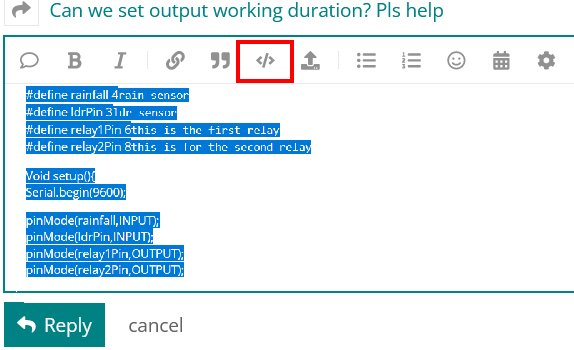# Error Help and Displaying Values Simulataneously

So I made a digital tach that's supposed to simultaneously read and display two variables. However, in my display protocol, I ran into this error.

"too few arguments to function 'void displayRPM(int, int)' "

//initialize LCD
#include <LiquidCrystal.h>
LiquidCrystal lcd(7,8,9,10,11,12);

//initialize hallSensor1 and its LED
const int hallSensor1=6; //hallSensor1
const int ledStat1=5; //ledStat1
int rpmMax1=0;

//intialize hallSensor2 and its LED
const int hallSensor2=4; //hallSensor2
const int ledStat2=3; //ledStat2
int rpmMax2=0;

//intialize common variables
const unsigned long initTime=1000; //initializ sampling time
const int maxRPM=1260; //arbitrary max rpm to be updated

void setup() {

//first system
pinMode(hallSensor1,INPUT_PULLUP); // hallSensor1 input_pullup
pinMode(ledStat1,OUTPUT); //output LED 1

//second system
pinMode(hallSensor2,INPUT_PULLUP); // hallSensor2 input_pullup
pinMode(ledStat2,OUTPUT); //output LED 2

//initialize LCD
lcd.begin(16,2);

//initialize Serial

}

//hallSensor1 RPM calculations
int getRPM1()
{
int count1=0;
boolean countFlag1=LOW; //initialize countFlag for On/Off
unsigned long countingTime1=0; //initialize countingTime to be zero
unsigned long beginTime1=millis(); //initialize beginTime;

while (countingTime1 <= initTime) //begin monitoring
{

if (digitalRead(hallSensor1)==HIGH) //if hallSensor1 is unswitched
{
digitalWrite(ledStat1,LOW); //LED is off
countFlag1=HIGH; //countFlag is on
}

if (digitalRead(hallSensor1)==LOW &&countFlag1==HIGH) //if hallSensor1 is switched
{
digitalWrite(ledStat1,HIGH); //LED is on
count1++; //update count
countFlag1=LOW; //countFlag is off
}

countingTime1=millis()-beginTime1;
}

int countRPM1= int(60000/float(initTime))*count1;
return countRPM1;

}

//hallSensor2 RPM calculations
int getRPM2()
{
int count2=0;
boolean countFlag2=LOW; //initialize countFlag for On/Off
unsigned long countingTime2=0; //initialize countingTime to be zero
unsigned long beginTime2=millis(); //initialize beginTime;

while (countingTime2 <= initTime) //begin monitoring
{

if (digitalRead(hallSensor2)==HIGH) //if hallSensor1 is unswitched
{
digitalWrite(ledStat2,LOW); //LED is off
countFlag2=HIGH; //countFlag is on
}

if (digitalRead(hallSensor2)==LOW &&countFlag2==HIGH) //if hallSensor1 is switched
{
digitalWrite(ledStat2,HIGH); //LED is on
count2++; //update count
countFlag2=LOW; //countFlag is off
}

countingTime2=millis()-beginTime2;
}

int countRPM2= int(60000/float(initTime))*count2;
return countRPM2;

}

void loop()
{
// display RPM1
int RPM1=getRPM1(); //rename updated variable to ease calculation

if (RPM1>rpmMax1)
{
rpmMax1=RPM1; //update RPM1 max
}
lcd.clear();
displayRPM(RPM1); //display RPM1
//fill in here

//display RPM2
int RPM2=getRPM2();
if (RPM2>rpmMax2)
{
displayRPM(RPM2); //dispay RPM2
}

}

void displayRPM(int RPM1, int RPM2)
{

}

I would've done separate displays like voidRPM1 and voidRPM2 but there's a delay whereas I need it simultaneously. I can take care of the code in the void later.

``````displayRPM(RPM2); //dispay RPM2  calling with one argument

void displayRPM(int RPM1, int RPM2)  // function definition for 2 arguments
``````

The displayRPM function takes 2 arguments, RPM1 and RPM2. You provided only one argument, RPM2. You can write a function definition with the same name that takes only one argument. See overloading a function.

Read the forum guidelines to see how to properly post code and some good information on making a good post.
Use the IDE autoformat tool (ctrl-t or Tools, Auto format) before posting code in code tags.

You can go back and fix your original post by highlighting the code and clicking the </> in the menu bar.Please include the entire error message. It is easy to do. There is a button (lower right of the IDE window) called "copy error message". Copy the error and paste into a post in code tags. Paraphrasing the error message leaves out important information.

Should be obvious. You defined the function to require TWO parameters, but when you call that function, you ONLY supply one parameter.

This change to loop() will fix your error message:

``````void loop()
{
// display RPM1
int RPM1 = getRPM1(); //rename updated variable to ease calculation

if (RPM1 > rpmMax1)
{
rpmMax1 = RPM1; //update RPM1 max
}

//display RPM2
int RPM2 = getRPM2();
if (RPM2 > rpmMax2)
{
rpmMax2 = RPM2; //update RPM2 max
}

lcd.clear();
displayRPM(RPM1, RPM2); //display RPM1 and RPM2
}``````

This topic was automatically closed 180 days after the last reply. New replies are no longer allowed.